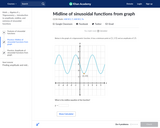Updating search results...

# 56 Results

View
Selected filters:
• NC.M3.F-IF.7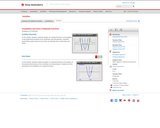Rating
0.0 stars

Students relate the graph of a rational function to the graphs of the polynomial functions of its numerator and denominator. Students graph these polynomials one at a time and identify their y-intercepts and zeros.

Subject:
Mathematics
Material Type:
Activity/Lab
Provider:
Texas Instruments
03/07/2018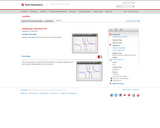Rating
0.0 stars

Students split rational functions into sums of partial fractions.

Subject:
Mathematics
Material Type:
Activity/Lab
Provider:
Texas Instruments
03/07/2018Rating
0.0 stars

In this lesson, students explore relationships between x-intercepts, factors, and roots of polynomial functions using the graphing calculator. Students also investigate rational functions, identifying the roots and the asymptotes as well as holes in the graphs. Students construct boxes of various dimensions using graph paper, collect height and volume data, and create a scatterplot in order to determine the height of the box with the maximum volume. Students can solve this problem using a graphing calculator or by using their own scatterplots drawn by hand. The use of questioning by the teacher, and the group work of the students are important features of this lesson.

Subject:
Math 1
Math 2
Math 3
Mathematics
Material Type:
Activity/Lab
Lesson Plan
Provider:
PBS
Author:
PBS Teachers Mathline
02/26/2019Rating
0.0 stars

Module includes tasks to conduct on graphing calculator regarding continuity, types of discontinuities and piecewise functions.

Subject:
Mathematics
Material Type:
Activity/Lab
Provider:
Texas Instruments
03/07/2018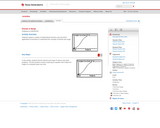Rating
0.0 stars

Students explore a variety of mathematical functions and real world applications of functions to understand the concepts of domain and range.

Subject:
Mathematics
Material Type:
Activity/Lab
Provider:
Texas Instruments
03/05/2018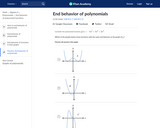Rating
0.0 stars

In this Khan Academy activity, students will determine what the end behavior of a polynomial looks like.

Subject:
Mathematics
Material Type:
Activity/Lab
Provider:
08/29/2018Rating
0.0 stars

In this Khan Academy activity, students will practice evaluating piecewise functions.

Subject:
Mathematics
Material Type:
Activity/Lab
Provider:
08/27/2018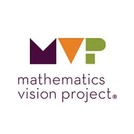Conditional Remix & Share Permitted
CC BY-NC-SA
Rating
0.0 stars

The purpose of this task is to build on students’ understanding of a logarithmic function as the inverse of an exponential function and their previous work in determining values for logarithmic expressions to find the graphs of logarithmic functions of various bases. Students use technology to explore transformations with log graphs in base 10 and then generalize the transformations to other bases.

Subject:
Math 3
Mathematics
Material Type:
Lesson
Author:
The Mathematics Vision Project
03/13/2020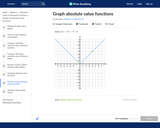Rating
0.0 stars

In this Khan Academy activity, students will graph absolute value functions.

Subject:
Mathematics
Material Type:
Activity/Lab
Provider:
08/29/2018Rating
0.0 stars

In this Khan Academy activity, students will graph a sinusoidal function.

Subject:
Mathematics
Material Type:
Activity/Lab
Provider:
09/04/2018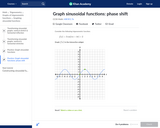Rating
0.0 stars

In this Khan Academy activity, students will graph a sinusoidal function with a phase shift.

Subject:
Mathematics
Material Type:
Activity/Lab
Provider:
09/04/2018Rating
0.0 stars

In this Khan Academy activity, students will graph exponential growth and decay problems.

Subject:
Mathematics
Material Type:
Activity/Lab
Provider:
09/04/2018Rating
0.0 stars

Students explore the key features of the graphs of rational functions and use the intercepts and asymptotes to create graphs of rational functions without using technology

Subject:
Mathematics
Material Type:
Lesson Plan
Provider:
EngageNY
02/02/2018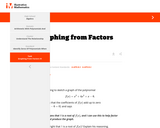Conditional Remix & Share Permitted
CC BY-NC-SA
Rating
0.0 stars

The task has students use the remainder theorem to deduce a linear factor of a cubic polynomial, and then to completely factor the polynomial. Students will need some procedure (e.g., synthetic or long division, or guess-and-check the coefficients) for determining the quadratic factor. Having the factored form permits students to deduce much about the structure of the graph.

Subject:
Mathematics
Material Type:
Activity/Lab
Provider:
Illustrative Mathematics
03/28/2018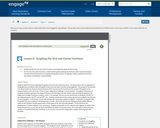Rating
0.0 stars

Students discover the key features of the sine and cosine functions and to connect the graphs to the measurements on the unit circle.

Subject:
Mathematics
Material Type:
Lesson Plan
Provider:
EngageNY
02/02/2018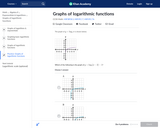Rating
0.0 stars

In this Khan Academy activity, students will graph logarithmic functions with transformations.

Subject:
Mathematics
Material Type:
Activity/Lab
Provider:
09/04/2018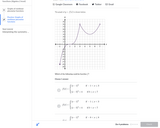Rating
0.0 stars

In this Khan Academy activity, students will use the graph of a non-linear piecewise function to determine the function.

Subject:
Mathematics
Material Type:
Activity/Lab
Provider:
08/29/2018Conditional Remix & Share Permitted
CC BY-NC-SA
Rating
0.0 stars

The purpose of this task is for students to surface comparisons between polynomials and whole numbers and use these comparisons to add and subtract polynomials algebraically. Students will also add and subtract polynomials given only graphically, adding corresponding points on the two graphs to obtain a sum. Students will make and test conjectures about the sum and differences of polynomials, such as “the sum of two quadratics is quadratic.”

Subject:
Math 3
Mathematics
Material Type:
Lesson
Author:
The Mathematics Vision Project
03/13/2020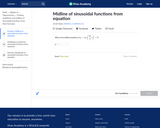Rating
0.0 stars

In this Khan Academy activity, students will identify the midline of sinusoidal functions from an equation.

Subject:
Mathematics
Material Type:
Activity/Lab
Provider:
09/04/2018